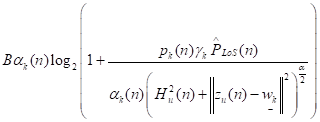# Cvx form for R=a(log2(1+b*c/a*d))?

`R=a(log2(1+b*c/a*d))`
in above equation “a” and “b” are variables.
i write this equation in cvx but could not run.
is this equation ok for above?
`-rel_entr((a*d),((a*d)+(b*c)))/log(2)`
zaheer

If you tried putting in some “random” numbers for the variables, you would see that is not equivalent to your original formula.

`-rel_entr(a,a+b*c/d)/log(2)` or `-1/d*rel_entr(a*d,a*d+b*c)/log(2)` will work.

dear Mark,
if `R=B*alpha*log2(1+power*gama0*pathloss/alpha*distance)` how i will write it in cvx, is it true or not as
`-rel_entr((alpha*distance),((alpha*distance)+(power*gama0*pathloss)))/log(2)`??
if not plz suggest me another accurate equation.
moreover, alpha, power are variable in it. plz suggest me. thanks

If you do it that way, you need to divide `-rel_entr(...)` by `distance`.

`-rel_entr(alpha,alpha+power*gama0*pathloss/distance)/log(2)`, which is simpler.

And of course, you need to multiply `-rel_entr(....)` by `B` to get `R`.

When making these reformulations, it is always a good idea to check by using random values (not special values such as 0 or 1) for the inputs to make sure the reformulation matches the original expression to within roundoff error. You should do that to make sure I didn’t make a mistake.

thanks, and B will be multiplied like this;
B(-rel_entr(alpha,alpha+powergama0pathloss/distance)/log(2))?

Yes. Or you can put the `B` between `-` and `rel_entr` so as to avoid use of a pair or parentheses.

humble thanks dear Mark

Of course you need `* ` between `B` and `rel_entr`, which I neglected to mention in my previous post.

yes i used it, without * it does not work, thanks

Good morning Mark,
i did this
`-St_Data.B*rel_entr(alpha1,alpha1+Temp_N/Temp_D)/log(2)`
but got this
Error using * (line 41)
Inner matrix dimensions must agree.

Error in / (line 15)
z = mtimes( x, y, ‘rdivide’ );

whats the problem. even all the terms in above code line are 1x10, only B is scalar.

If `Temp_N` and `Temp_D` are both 1 by 10, you need `Temp_N./Temp_D`

Perhaps you need to brush up on basic MATLAB.

as i did this
Temp_N./Temp_D
this is the result
Error using .* (line 173)
Disciplined convex programming error:
Cannot perform the operation: {real affine} ./ {real affine}

Error in ./ (line 19)
z = times( x, y, ‘./’ );
i did this
times(Temp_N,Temp_D,‘./’)
to resolve error of (line19)
then found this
Error using .* (line 173)
Disciplined convex programming error:
Cannot perform the operation: {real affine} ./ {real affine}
now my question is how to resolve this issue of {real affine} ./ {real affine}.

dear Mark i don’t know why this error come.
plz suggest me to resolve such issues, before this i also got much help from your comments regarding cvx.

i have stuck at this point,
even i have checked all possible ways as i know
-1*inv_pos(Temp_D).St_Data.B.rel_entr(alpha1,alpha1+Temp_N)/log(2)
but
Error using .
(line 173)
Disciplined convex programming error:
Cannot perform the operation: {concave} .
{convex}

-St_Data.B*rel_entr((Temp_D),((Temp_D)+(Temp_N)))/log(2)

ans =

``````cvx concave expression (1x10 vector)
``````

this type give me ok report, where Temp_D, Temp_N are 1x1o matrices while B is scalar.
what do you say it’s enough or i have to divide the whole term with Temp_D?

i show you my original equation to whome i have to code in cvx matlab.where B is bandwidth, alpha, pk are decision variables, Hu is UAV altitude zu is UAV position, wk is user position. Plos_hat is pathloss which is constant here.
now please suggest me how to code in cvx matlab.

The formulation I provided earlier was valid based on what you said were the variables and constants. When you then showed an expression involving `Temp_D`, I assumed it was a vector version of the earlier expression, and therefore `Temp_D` is a constant, which it’s not. Hence the error message.

As for the expression in the most recent post, I have no reason to think it’s either concave or convex, due to the `(...)^(alpha/2)`, which is an exponential. If that expression is concave, you need to prove it.

For your future reference, please note the requirements on the arguments of `rel_entr`.

help rel_entr

`````` rel_entr   Scalar relative entropy.
rel_entr(X,Y) returns an array of the same size as X+Y with the
relative entropy function applied to each element:
{ X.*LOG(X./Y) if X >  0 & Y >  0,
rel_entr(X,Y) = { 0            if X == 0 & Y >= 0,
{ +Inf         otherwise.
X and Y must either be the same size, or one must be a scalar. If X and
Y are vectors, then SUM(rel_entr(X,Y)) returns their relative entropy.
If they are PDFs (that is, if X>=0, Y>=0, SUM(X)==1, SUM(Y)==1) then
this is equal to their Kullback-Liebler divergence SUM(KL_DIV(X,Y)).
-SUM(rel_entr(X,1)) returns the entropy of X.

Disciplined convex programming information:
rel_entr(X,Y) is convex in both X and Y, nonmonotonic in X, and
nonincreasing in Y. Thus when used in CVX expressions, X must be
real and affine and Y must be concave. The use of rel_entr(X,Y) in
an objective or constraint will effectively constrain both X and Y